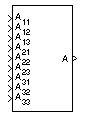Create 3x3 Matrix

Create 3-by-3 matrix from nine input values

• Library:
• Aerospace Blockset / Utilities / Math Operations

•Description

The Create 3x3 Matrix block creates a 3-by-3 matrix from nine input values where each input corresponds to an element of the matrix.

The output matrix has the form of

$A=\left[\begin{array}{ccc}{A}_{11}& {A}_{12}& {A}_{13}\\ {A}_{21}& {A}_{22}& {A}_{23}\\ {A}_{31}& {A}_{32}& {A}_{33}\end{array}\right]$

Ports

Input

expand all

First row, first column of the matrix, specified as a matrix element.

Example: 1

Data Types: double

First row, second column of the matrix, specified as a matrix element.

Example: 2

Data Types: double

First row, third column of the matrix, specified as a matrix element.

Example: 3

Data Types: double

Second row, first column of the matrix, specified as a matrix element.

Example: 4

Data Types: double

Second row, second column of the matrix, specified as a matrix element.

Example: 5

Data Types: double

Second row, third column of the matrix, specified as a matrix element.

Example: 6

Data Types: double

Third row, first column of the matrix, specified as a matrix element.

Example: 7

Data Types: double

Third row, second column of the matrix, specified as a matrix element.

Example: 8

Data Types: double

Third row, third column of the matrix, specified as a matrix element.

Example: 9

Data Types: double

Output

expand all

Matrix, output as a 3-by-3 matrix.

Data Types: double

Extended Capabilities

C/C++ Code GenerationGenerate C and C++ code using Simulink® Coder™.